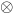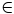The Australian Journal of Mathematical Analysis and Applications

 Home News Editors Volumes RGMIA Subscriptions Authors Contact

ISSN 1449-5910

Paper Information

Paper Title:

On the Convergence in Law of Iterates of Random-Valued Functions

Author(s):

Karol Baron

Uniwersytet Śląski, Instytut Matematyki
Bankowa 14, PL--40--007 Katowice,
Poland
baron@us.edu.pl

Abstract:

Given a probability space (Ω, A, P) a separable and complete metric space X with the σ-algebra B of all its Borel subsets and a BA -measurable f : X * Ω → X we consider its iterates fn, nN, defined on X * ΩN by f1(x,ω) = f(x,ω1)  and fn+1(x,ω)=f(fn(x,ω),ωn+1), provide a simple criterion for the convergence in law of fn(x,·)) nN, to a random variable independent of xX , and apply this criterion to linear functional equations in a single variable.

Full Text PDF: